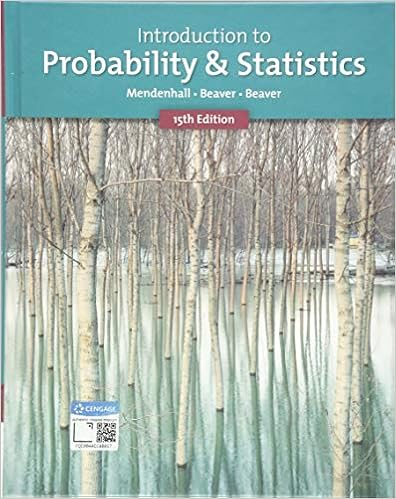# Given a different feed supplement you are to analyze

• Homework Help
• 2
• 100% (3) 3 out of 3 people found this document helpful

This preview shows page 1 - 2 out of 2 pages.

##### We have textbook solutions for you!
The document you are viewing contains questions related to this textbook.The document you are viewing contains questions related to this textbook.
Chapter 13 / Exercise 11
Introduction to Probability and Statistics
Beaver/MendenhallExpert Verified
given a different feed supplement. You are to analyze how the weight (grams, after six weeks) of a chick depends on the feed that it was assigned. (a) [2 pts] Fit an appropriate ANOVA model. Display a summary of your results. 1 Even though sex is a categorical variable, it can be used as a numerical predictor here because it has only two levels, and they are numerically encoded.
1
##### We have textbook solutions for you!
The document you are viewing contains questions related to this textbook.The document you are viewing contains questions related to this textbook.
Chapter 13 / Exercise 11
Introduction to Probability and Statistics
Beaver/MendenhallExpert Verified
(b) [2 pts] Produce an ANOVA table. (c) [2 pts] Test whether there are any differences among the mean weights of the groups (based on an F -test at α = 0 . 05). (d) [2 pts] Produce Tukey simultaneous 95% confidence intervals for all mean differences between pairs of groups. (e) [2 pts] According to your Tukey intervals, which pairs of feeds have significantly different means (after adjusting for multiple comparisons)? (f) [2 pts] Produce the usual diagnostic plots for your model, and draw conclusions. 4. [GRADUATE STUDENTS ONLY] The data set infmort contains data on infant mortality in 105 nations, 2 along with their per capita income and a factor indicating whether or not they export oil. (a) [2 pts] Fit the regression of log(mortality) on log(income) and oil . Give a summary of the results. Is oil significant? (b)
•••